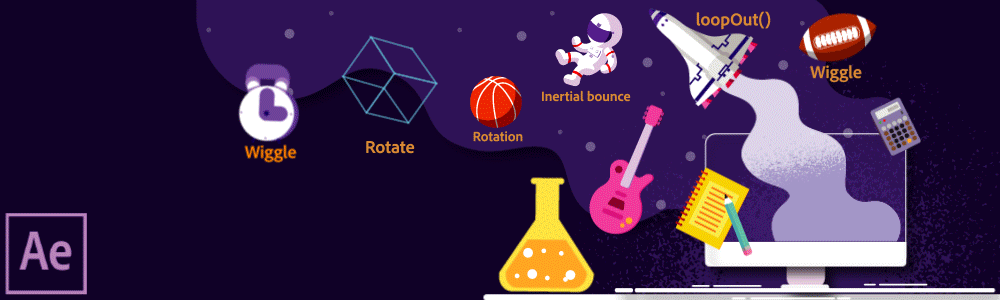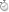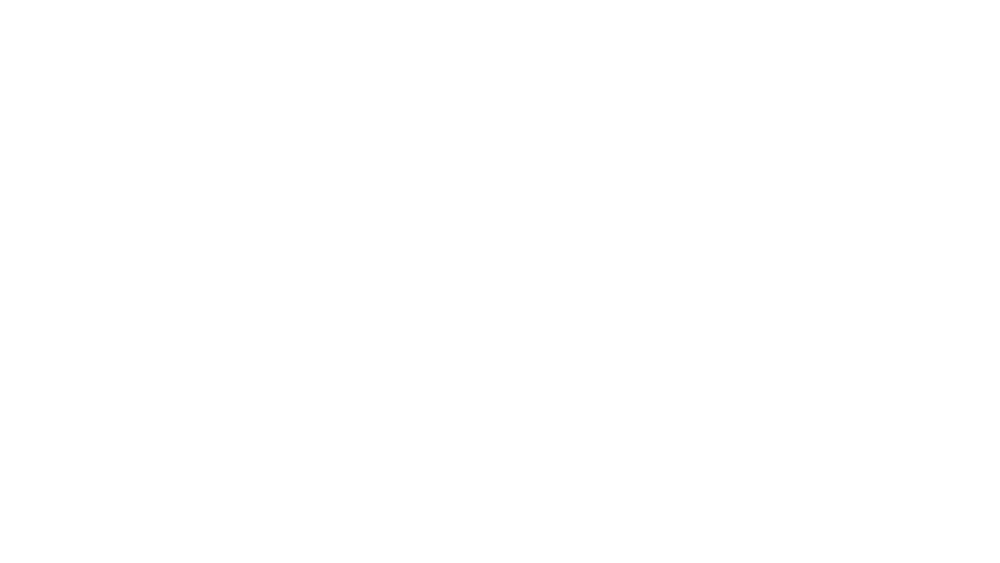User Guide Cancel

# Expression examples## Wiggle randomly

The wiggle expression is one of the most common After Effects expressions. It wiggles an object across random values. This expression can be used to make your scene seem more natural. You add this expression to the Position property of the layer.

1. Select a layer in your composition.

2. Press P to reveal its Position property in the Timeline panel.

3. Alt-click (Windows) or Option-click (macOS) the stopwatch to the left of the property name.

4. Enter the following code snippet in the expression field:

`wiggle(2,30)`

In this example, wiggle (frequency, amount)where the first number is the number of wiggles per second and the second number is the value of the wiggle. So, using wiggle(2,30)will make the layer wiggle 2 times per second up to 30 pixels in any direction.

## Control a wiggle with Slider controls

Expressions can be keyframed by replacing values with links to expression controls, like a Slider Control. By replacing the second argument to the wiggle() expression with a link to a Slider Control, you can keyframe the behavior to start and stop at specific times.

1. Add a Slider Control effect to any Layer and name it "Wiggle Amount".

2. Apply the following expression to the Position property of the same layer:

```// Uses the pick-whip to create the "effect(...)" link to the Slider
var wiggleAmount = effect("Wiggle Amount")("Slider");

// Wiggles 4 times per second by the amount set by the Slider
wiggle( 4, wiggleAmount );```

## Layer revolves in a circle

You can create an expression without using properties from other layers. For example, you can make a layer revolve around the center of the composition.

1. Select a layer in your composition.

2. Press P to reveal its Position property in the Timeline panel.

3. Alt-click (Windows) or Option-click (macOS) the stopwatch to the left of the property name.

4. Enter the following code snippet in the expression field:

```var centerOfComp = [ ( thisComp.width / 2 ), ( thisComp.height / 2) ];
var circleOverTime = [ Math.sin( time ) * 50, -Math.cos( time ) * 50 ];
centerOfComp + circleOverTime;```

## Overshoot

Commonly known as inertial bounce, this expression uses the animation of a layer's own keyframes to create a natural overshoot. It creates a bouncing motion of any parameter from one keyframe to the next, based on its velocity. The bounce happens in whatever direction the object is traveling. To achieve this animation:

1. Create or import your graphics in After Effects.

2. Add keyframes to the Position property of the layer that you want to animate.

3.  Add the following expression to the Position property of the layer:

```// Sets up values to control overshoot.
// Link these to Slider expression controls to quickly preview different settings.
var amp = 40;
var freq = 30;
var decay = 50;

// Finds the most recent keyframe
var nK = nearestKey(time);
var n = (nK.time <= time) ? nK.index : --nK.index;
var t = (n === 0) ? 0 : time - key(n).time;

// If the current time is later than a keyframe, calculate overshoot.
// If not, use original value.
if ( n > 0 && t < 1 ) {
var v = velocityAtTime( key( n ).time - thisComp.frameDuration /10 );
value + v * amp * .001 * Math.sin(freq * .1 * t * 2 * Math.PI) / Math.exp(decay * .1 * t);
} else {
value;
}```

## Rotate with time

You can use the pick whip to link rotation values between layers to animate objects. The way a clock works, consider these three circles as three hands of the clock - the hour hand moves from hour to hour, the minute hand rotates the full circumference of the clock face.

1. Import or create three circles solid-color layers. Let's assume one of them works like an hour hand, the other one as the minute hand, and the third as the seconds hand. (See Solid-color layers and solid-color footage items.

2. Set the anchor points at the ends of the layers. (See Layer anchor points.)

3. Move the layers so that the anchor points are at the center of the composition. (See Move layers in space.)

4. Set Rotation keyframes for the hour hand. (See Set or add keyframes), select the Rotation property for the minute hand, and choose Animation > Add Expression.

5. Drag the pick whip to the Rotation property for the biggest circle. The following expression appears:

` thisComp.layer("circle").rotation`
6. To make the second circle rotate 12 times as fast as the first one, add *12 at the end of the expression as follows:

`thisComp.layer("circle").rotation*12`
7. Repeat the same with the third circle and add *24 at the end of the expression:

`thisComp.layer("circle").rotation*24`

## Loop

Expressions can be used to loop and extend animation without adding additional keyframes - for example, multiple shapes can be made to spin until the end of a composition. To achieve this, add a keyframe to the Rotation property for the starting rotation and then another with the ending rotation. Applying the loopOut()method to the keyframed Rotation property will then allow the layer to keep spinning after the last keyframe.

The arguments used in the loopOut()example below set the type of loop and how many keyframes to include in the loop.

```//loopOut set to cycle all keyframes
loopOut("cycle", 0);```

The first argument is "cycle", one of four available loop modes for the loopOut method. The other three are "continue", "offset", and "ping-pong""cycle" begins to loop at the last keyframe, starting again at the values of the first keyframe in the range defined by the second argument.

The second argument is the number of keyframes to include in the loop, counted backward from the last keyframe. If the second argument isn't given or is set to 0, all keyframe animation on the property will be looped after the last keyframe. If the argument is 1, then the animation between the last keyframe and the one before it will be looped. If the argument is 2, then the looped animation will be between the last keyframe and the two keyframes before it, and so on.

## Get the true Position of a parented layer

When a layer is parented, the value shown in its Position property is not its "true" location in the Composition but is instead relative to its parent layer's location. To find the parented layer's true location, an expression must be used to convert the parent layer's coordinate space to the composition's coordinate space. Apply the following expression to the Position of an unparented layer to link it to the location of parented layer:

```// Defines the parented layer
var targetLayer = thisComp.layer("Parented Layer");

// Finds the parented layer's Anchor Point in the Composition
targetLayer.toComp( targetLayer.anchorPoint );```

## Delay a layer's Position from its parent

Delay and offset are great ways to make animation more dynamic and natural. You can create and control these types of offsets by parenting many layers to one parent layer and then applying an expression to the Position of each child to delay the motion inherited from the parent.

Note this will only work with parented layers.

```// Sets a delay amount in frames
var delay = 5;

// Offsets the layer's Position in time based on delay
parent.fromComp( toComp( anchorPoint, time - framesToTime( delay ) ) );```

You can also drive the delay amount based on the index of the child layer relative to the parent layer. This allows you to easily reorder the animation of the child layers by reordering them in the Timeline below the parent (or above if you want their animation to happen before the parent ) :

```// Sets a delay amount in frames
var delay = 5;

// Multiplies delay based on this layer's index relative to it's parent
var multiplyDelay = delay * ( index - parent.index )

// Offsets layer's Position in time based on delay
parent.fromComp( toComp( anchorPoint, time - framesToTime( multiplyDelay ) ) );```

## Expression example: Start or stop wiggle at specific time

Add the following expression to the Position property of an element to initiate a wiggle effect starting 2 seconds into the animation:

```var timeToStart = 2;

if (time > timeToStart) {
wiggle(3,25);
} else {
value;
}```

Add the following expression to the Position property of an element to initiate a wiggle effect at the beginning of the animation and stop after 4 seconds:

```var timeToStop = 4;

if ( time > timeToStop ) {
value;
} else {
wiggle( 3, 25 );
}```

Add the following expression to the Position property of an element to initiate a wiggle effect at the beginning after 2 seconds of the animation and stop after 4 seconds:

```var timeToStart = 2;
var timeToStop = 4;

if ( ( time > timeToStart ) && ( time < timeToStop ) ) {
wiggle(3,25);
} else {
value;
}```

## Expression example: Position one layer between two others

This example expression positions and maintains one layer at a balanced distance between two other layers.

2. Animate the positions of the first two layers in the Timeline panel. (See Motion paths.)

3. Select the third layer. Press P to reveal the Position property. Alt-click (Windows) or Option-click (macOS) the stopwatchbutton to the left of the property name.

4. Enter the following in the expression field:
`( thisComp.layer(1).position + thisComp.layer(2).position ) / 2`

## Create a trail of imagesThis example expression instructs a layer to be at the same position as the next higher layer in the Timeline panel, but delayed by a specified amount of time (in this case, 0.5 seconds). You can set similar expressions for the other geometric properties.

1. Start with a shape layer scaled to approximately 30% of the composition size. (See Solid-color layers and solid-color footage items.)

2. Open the Position property and add keyframes. Select the layer. Press P to reveal the Position property. Alt-click (Windows) or Option-click (macOS) the stopwatchbutton to the left of the property name. (See Setting, selecting, and deleting keyframes.)

3. Enter the following in the expression field:

`thisComp.layer(thisLayer, -1).position.valueAtTime(time - .5)`
4. Duplicate the last layer five times by selecting it and pressing Ctrl+D (Windows) or Command+D (macOS) five times. All layers follow the same path, and each is delayed 0.5 seconds from the previous.

## Expression example: Create a bulge between two layers

This example expression synchronizes the Bulge Center argument of the Bulge effect in one layer with the position of another layer. For example, you can create an effect that looks like a magnifying glass moving over a layer, with the contents under the magnifying glass bulging as the lens (that is, the overlying layer) moves. This expression uses the fromWorld method, which makes the expression work correctly regardless of whether you move the magnifying glass layer or the underlying layer. You can rotate or scale the underlying layer, and the expression stays intact.

You can also use other effects, such as Ripple, with this expression.

1. Start with two layers. Make one layer a magnifying glass or similar object with a hole in the middle and name it 'Magnifier'. (See Creating layers.)

2. Animate the position of the magnifying glass layer. (See Motion paths.)

3. Apply the Bulge effect to the other layer. (See Apply an effect or animation preset.)

4. Select the Bulge Center property of the Bulge effect in the Timeline panel. choose Animation > Add Expression, or Alt-click (Windows) or Option-click (macOS) the stopwatchbutton for the property.

5. Select the default expression text and type the following:
`fromWorld(thisComp.layer("Magnifier").position)`

## Expression example: Fade opacity of a 3D layer based on distance from camera

Apply the following expression to the Opacity property of a 3D layer:

```var  startFade = 500; // Start fade 500 pixels from camera.

try {
// Check whether there's a camera
var C = thisComp.activeCamera.toWorld([0,0,0]);
} catch ( err ) {
// No camera, so assume 50mm
var w = thisComp.width * thisComp.pixelAspect;
var C = [0,0,-z];
}

var P = toWorld( anchorPoint );
var d = length( C, P );

The fade starts at a distance of 500 pixels from the camera and is complete at 1500 pixels from the camera. The linear interpolation method is used to map distance values to opacity values.

## Expression example: Make a 3D layer invisible if facing away from camera

Apply the following expression to the Opacity property of a 3D layer:

```if ( toCompVec([0, 0, 1]) > 0 ) {
value;
} else {
0;
}```

## Expression example: Flip layer horizontally if facing away from camera

Apply the following expression to the Scale property of a 3D layer:

```if ( toCompVec([0, 0, 1]) > 0 ) {
value;
} else {
[ -value, value, value ];
}```

## Expression example: Animate scale at each layer marker

Apply the following expression to a Scale property to make a layer wobble at each marker:

```var n = 0;
var t = 0;

if (marker.numKeys > 0){
n = marker.nearestKey(time).index;
if (marker.key(n).time > time) n--;
}
if (n > 0) t = time - marker.key(n).time;

var amp = 15;
var freq = 5;
var decay = 3.0;

var angle = freq * 2 * Math.PI * t;
var scaleFact = (100 + amp * Math.sin(angle) / Math.exp(decay * t)) / 100;

[value * scaleFact, value / scaleFact];```
Note:

To add a marker, select Layer > Markers > New Marker.

You can use any expression in place of the wiggle expression used here, to begin and end the influence of any expression at a specific time.

## Expression example: Match camera focal plane to another layer

Apply the following expression to the Focus Distance property of a camera layer to have its focus distance match the distance to the anchor point of a layer named 'Target':

```var target = thisComp.layer("target");
var V1 = target.toWorld( target.anchorPoint ) - toWorld( [ 0, 0, 0 ] );
var V2 = toWorldVec( [ 0, 0, 1 ] );

dot( V1, V2 );```

Dan Ebberts explains this expression example in detail on his Motionscript website.

## More Expression resourcesNow that you have understood some of the concepts behind expressions, come to the community for some real-life examples, and to share your work.

You can also check out Dan Ebberts' excellent collection of example expressions and tutorials on his MotionScript website.

Dan Ebberts provides example expressions and tutorials for learning how to work with expressions on his MotionScript website. For example, Dan provides an excellent page about collision detection.

## More expression examples

Rick Gerard provides an example on his website that demonstrates rolling a square object along a floor so that the sides stay in contact with the floor plane.

Colin Braley provides a tutorial and example project on his website that show how to use expressions to make one layer repel others in a natural-seeming manner.

The AE Enhancers forum provides many examples and much useful information about expressions, as well as scripts and animation presets. In this post on the AE Enhancers forum, Paul Tuersley provides a tutorial and example project that show how to use expressions to animate several layers in a swarm.

### More like this